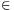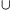Mathematical and Physical Journal
for High Schools
Issued by the MATFUND Foundation
 Already signed up? New to KöMaL?

#Problem A. 382. (October 2005)

A. 382. S and T are disjoint sets, * is a binary operation on the elements of S and o is a binary operation on the elements of T. (That is, if a,bS and c,dT, then a*bS and codT). Each operation is associative. In other words, (S,*) and (T,o) are semigroups. It is also given that for every tT there are elements u,vT, such that uot=tov=t. Letdenote an arbitrary mapping. Define the operationon the set ST as follows:Show that the operationis associative if and only if f is a homomorphism, that is, f(a*b)=f(a)of(b) for all a,bS.

Czech competition problem

(5 pont)

Deadline expired on November 15, 2005.

Solution. (a) If f is a homomorphism thenis trivially associative.

(b) Suppose thatis associative and let a,b be two arbitrary elements of S. By the conditions there exist u,vT such that uof(a)=f(a) and f(a*b)ov=f(a*b). Then### Statistics:

 15 students sent a solution. 5 points: Erdélyi Márton, Estélyi István, Fischer Richárd, Gyenizse Gergő, Hujter Bálint, Jankó Zsuzsanna, Kónya 495 Gábor, Korándi Dániel, Nagy 224 Csaba, Paulin Roland. 4 points: Kisfaludi-Bak Sándor, Tomon István. 2 points: 2 students. 1 point: 1 student.

Problems in Mathematics of KöMaL, October 2005